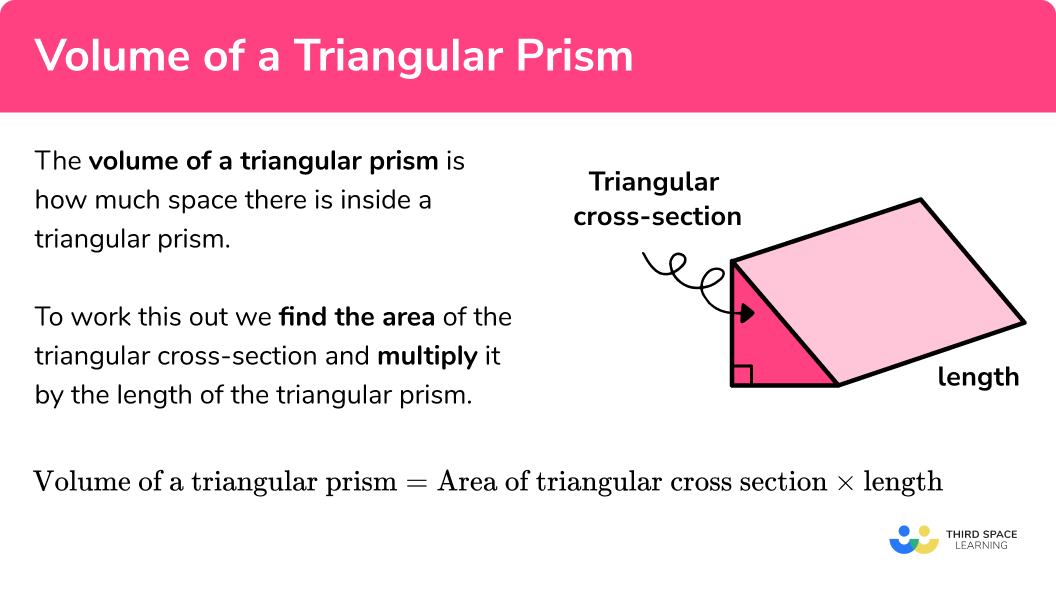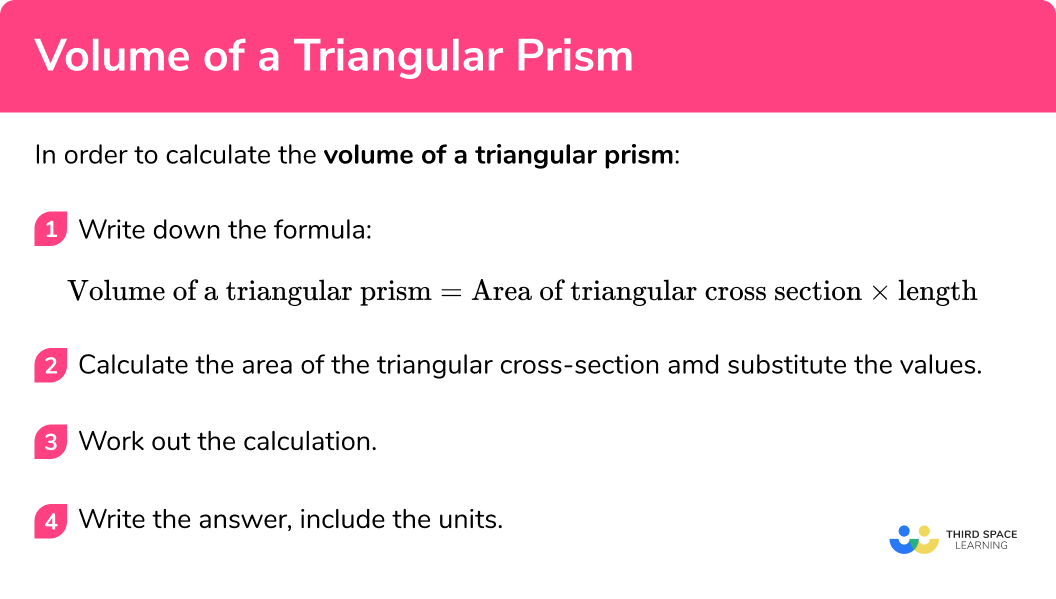# Volume Of A Triangular Prism

Here we will learn about the volume of a triangular prism, including how to calculate the volume and how to find a missing length given the volume.

There are also volume and surface area of a triangular prism worksheets based on Edexcel, AQA and OCR exam questions, along with further guidance on where to go next if you’re still stuck.

## What is volume of a triangular prism?

The volume of a triangular prism is how much space there is inside a triangular prism. A triangular prism is a polyhedron (3D shape made from polygons) with two congruent triangular ends connected by three rectangles.

To work this out we find the area of the triangular cross-section and multiply it by the length.

Volume of a triangular prism = Area of triangular cross section x length

E.g.

$\begin{array}{l} \text{Area of triangular cross-section:}\\ \text{Area }=\frac{1}{2}bh\\ \text{Area }=\frac{1}{2} \times 4 \times 5\\ \text{Area }=10\mathrm{cm}^{2}\\ \\ \text{Volume of triangular prism:}\\ \text{Volume }= \text{Area of triangular cross-section } \times \text{ length}\\ \text{Volume }=10 \times 11\\ \text{Volume }=110\mathrm{cm}^{3} \end{array}$

Volume is measured in cubic units (e.g. mm^3, cm^3, m^3 etc).

### What is volume of a triangular prism?## How to calculate the volume of a triangular prism

In order to calculate the volume of a triangular prism:

1. Write down the formula.
Volume of a triangular prism = Area of triangular cross section \times length
2. Calculate the area of the triangular cross-section and substitute the values.
3. Work out the calculation.
4. Write the answer, including the units.

### How to calculate the volume of a triangular prism## Volume of a triangular prism examples

### Example 1: volume of a triangular prism

Work out the volume of this triangular prism

1. Write down the formula.

Volume of a triangular prism = Area of triangular cross section x length

2Calculate the area of the triangular cross-section and substitute the values.

The base of the triangle is 2cm and the height of the triangle is 3cm .

$\begin{array}{l} \text{Area of triangle }=\frac{1}{2} \times b \times h\\ \text{Area of triangle }=\frac{1}{2} \times 2 \times 3\\ \text{Area of triangle }=3 \end{array}$

The area of the triangle is 3cm^2 .

The length of the prism is 7cm .

Volume of triangular prism = Area of triangular cross section x length

Volume of triangular prism = 3 × 7

3Work out the calculation.

Volume of triangular prism = 3 × 7

Volume of triangular prism = 21

4Write the answer, including the units.

The measurements on this triangular prism are in cm so the volume will be measured in cm^3 .

Volume = 21cm^3

### Example 2: triangular prism with isosceles triangle

Work out the volume of the triangular prism

Volume of a triangular prism = Area of triangular cross section x length

$\begin{array}{l} \text{Area of triangle }=\frac{1}{2} \times b \times h\\ \text{Area of triangle }=\frac{1}{2} \times 5 \times 8\\ \text{Area of triangle }=20 \end{array}$

Volume of triangular prism = Area of triangular cross section x length

Volume of triangular prism = 20 × 18

Volume of triangular prism = 20 × 18

Volume of triangular prism = 360

The measurements on this triangular prism are in mm so the volume will be measured in mm^3 .

Volume = 360mm^3

### Example 3: different units

Work out the volume of this triangular prism

Volume of a triangular prism = Area of triangular cross section x length

This time, the triangular prism is the other way up so we start by calculating the area of the base. There are some measurements in both m and cm here so we need to make the units the same before we begin calculating. The easiest thing to do in this example is to convert 0.1m to 10cm .

$\begin{array}{l} \text{Area of triangle }=\frac{1}{2} \times b \times h\\ \text{Area of triangle }=\frac{1}{2} \times 10 \times 10\\ \text{Area of triangle }=50 \end{array}$

Since the triangular prism is the other way up, the length that we need to multiply by is the height of the prism, 21cm .

Volume of triangular prism = Area of triangular cross section x length

Volume of triangular prism = 50 × 21

Volume of triangular prism = 50 × 21

Volume of triangular prism = 1050

The measurements that we used for this triangular prism are in cm so the volume will be measured in cm^3 .

Volume = 1050cm^3

## Calculating a missing length

Sometimes we might know the volume and some of the measurements of a triangular prism and we might want to work out the other measurements. We can do this by substituting the values that we know into the volume of a triangular prism formula and solving the equation that is formed.

## How to work out a missing length given the volume

In order to calculate the length given the volume:

1. Write down the formula.
Volume of a triangular prism = area of triangular cross section x length
2. Calculate the area of the triangular cross-section and substitute everything into the volume of a triangular prism formula.
3. Solve the equation.
4. Write the answer, include the units.

## Missing length examples

### Example 4: finding a missing length

The volume of this triangular prism is 168cm^3 . Work out the length, x ,  of the triangular prism.

Volume of a triangular prism = area of triangular cross section x length

$\begin{array}{l} \text{Area of triangle }=\frac{1}{2} \times b \times h\\ \text{Area of triangle }=\frac{1}{2} \times 7 \times 6\\ \text{Area of triangle }=21 \end{array}$

\begin{aligned} \text{Volume of a triangular prism } &= \text{ area of triangular cross-section } \times { length}\\ 168&=21 \times x \end{aligned}

\begin{aligned} 21x&=168\\ x&=8 \end{aligned}

x=8cm

### Example 5: finding a missing height

The volume of this triangular prism is 80mm^3 . Work out the height of the prism.

Volume of a triangular prism = area of triangular cross section x length

$\begin{array}{l} \text{Area of triangle }=\frac{1}{2} \times b \times h\\ \text{Area of triangle }=\frac{1}{2} \times 4 \times h\\ \text{Area of triangle }=2h \end{array}$

\begin{aligned} \text{Volume of a triangular prism } &= \text{ area of triangular cross-section } \times { length}\\ 80&=2h \times 16\\ 80 &= 32h \end{aligned}

\begin{aligned} 32h&=80\\ h&=2.5 \end{aligned}

x=2.5mm

### Example 6: finding a missing base, different units

The volume of this triangular prism is 440mm^2 . Work out the length labelled y .

Volume of a triangular prism = area of triangular cross section x length

$\begin{array}{l} \text{Area of triangle }=\frac{1}{2} \times b \times h\\ \text{Area of triangle }=\frac{1}{2} \times y \times 4\\ \text{Area of triangle }=2y \end{array}$

Notice here that we need to work in mm since the volume is in mm^3 . Therefore we need to convert 2cm to 20mm .

\begin{aligned} \text{Volume of a triangular prism } &= \text{ area of triangular cross-section } \times { length}\\ 440&=2y \times 20\\ 440 &= 40y \end{aligned}

\begin{aligned} 40y&=440\\ y&=11 \end{aligned}

y=11mm

### Common misconceptions

• Missing/incorrect units

Volume is measured in units cubed (e.g. mm^3, cm^3, m^3 etc)

• Calculating with different units

You need to make sure all measurements are in the same units before calculating volume.
E.g. you can’t have some in cm and some in m

• Using the wrong formula

Be careful to apply the correct prism related formula to the correct question type.

Volume of a triangular prism is part of our series of lessons to support revision on triangular prism. You may find it helpful to start with the main triangular prism lesson for a summary of what to expect, or use the step by step guides below for further detail on individual topics. Other lessons in this series include:

### Practice volume of a triangular prism questions

1. Work out the volume of the triangular prism110 \mathrm{cm}^{3}55 \mathrm{cm}^{3}240 \mathrm{cm}^{3}120 \mathrm{cm}^{3}\begin{aligned} \text{Area of triangle }&=\frac{1}{2} \times 3 \times 8\\ &=12\mathrm{cm}^{2} \end{aligned}

\begin{aligned} \text{Volume of triangular prism }&=12 \times 10\\ &=120\mathrm{cm}^{3} \end{aligned}

2. Work out the volume of the triangular prism252 \mathrm{cm}^{3}42 \mathrm{cm}^{3}1764 \mathrm{cm}^{3}126 \mathrm{cm}^{3}\begin{aligned} \text{Area of triangle }&=\frac{1}{2} \times 6 \times 6\\ &=18\mathrm{cm}^{2} \end{aligned}

\begin{aligned} \text{Volume of triangular prism }&=18 \times 7\\ &=126\mathrm{cm}^{3} \end{aligned}

3. Work out the volume of the triangular prism924 \mathrm{mm}^{3}9.24 \mathrm{cm}^{3}92.4 \mathrm{mm}^{3}9240 \mathrm{mm}^{3}Notice that one of the measurements is in mm and the others are in cm . We can change 1.4cm to 14mm and 2.2cm to 22mm .

\begin{aligned} \text{Area of triangle }&=\frac{1}{2} \times 14 \times 6\\ &=42\mathrm{mm}^{2} \end{aligned}

\begin{aligned} \text{Volume of triangular prism }&=42 \times 22\\ &=924\mathrm{mm}^{3} \end{aligned}

4. The volume of this triangular prism is 84cm^3 . Work out the length, x , of the triangular prism3.5cm2016cm7cm1008cm\begin{aligned} \text{Area of triangle }&=\frac{1}{2} \times 3 \times 8\\ &=24\mathrm{cm}^{2} \end{aligned}

\begin{aligned} \text{Volume of triangular prism }&=12 \times x\\ 84 &= 12x\\ 7&=x \end{aligned}

The length is 7cm .

5. The volume of this triangular prism is 405m^3 . Work out the height of the triangular prism45m9m2.5m4.5m\begin{aligned} \text{Area of triangle }&=\frac{1}{2} \times 6 \times h\\ &=3h \end{aligned}

\begin{aligned} \text{Volume of triangular prism }&=3h \times 15\\ 405 &= 45h\\ 9&=h \end{aligned}

The height is 9m .

6. The volume of this triangular prism is 45cm^3 . Work out the length of y .5cm0.25cm0.5cm4050cm\begin{aligned} \text{Area of triangle }&=\frac{1}{2} \times 4 \times y\\ &=4y \end{aligned}

Notice that the height of the triangular prism is in mm however the volume is in cm3. Therefore we need to change 45mm to 4.5cm .

\begin{aligned} \text{Volume of triangular prism }&=2y \times 4.5\\ 45 &= 9h\\ 5&=h \end{aligned}

The length of y is 5cm .

### Volume of a triangular prism GCSE questions

1. Work out the volume of the triangular prism.(2 marks)

\begin{aligned} \text{Area of triangle }&=\frac{1}{2} \times 5 \times 2\\ &=5 \mathrm{cm}^{2} \end{aligned}

(1)

\begin{aligned} \text{Volume of triangular prism }&=5 \times 6\\ &=30\mathrm{cm}^{3} \end{aligned}

(1)

2. These triangular prisms have the same volume. Work out the height, h , of prism B .(5 marks)

\begin{aligned} \text{Area of triangle A }&=\frac{1}{2} \times 8 \times 3\\ &=12 \mathrm{cm}^{2} \end{aligned}

(1)

\begin{aligned} \text{Volume of triangular prism A }&=12 \times 12\\ &=144\mathrm{cm}^{3} \end{aligned}

(1)

\begin{aligned} \text{Area of triangle B }&=\frac{1}{2} \times 4 \times h\\ &=2h \end{aligned}
\begin{aligned} \text{Volume of triangular prism B}&=2h \times 14.4\\ \end{aligned}

(1)

144 = 28.8h

(1)

h = 5cm

(1)

3. (a) Work out the volume of the triangular prism.(b) A section, 4cm tall, is cut off of the top of the triangular prism. Find the volume of the remaining shape.(5 marks)

(a)
\begin{aligned} \text{Area of triangle }&=\frac{1}{2} \times 5 \times 8\\ &=20 \mathrm{cm}^{2} \end{aligned}

(1)

\begin{aligned} \text{Volume of triangular prism }&=20 \times 15\\ &=300\mathrm{cm}^{3} \end{aligned}

(1)

(b)
\begin{aligned} \text{Area of small triangle }&=\frac{1}{2} \times 2.5 \times 4\\ &=5 \mathrm{cm}^{2} \end{aligned}

(1)

\begin{aligned} \text{Volume of small triangular prism }&=5 \times 15\\ &=75\mathrm{cm}^{3} \end{aligned}

(1)

\begin{aligned} \text{Volume of remaining shape }&=300-75\\ &=225\mathrm{cm}^{3} \end{aligned}

(1)

## Learning checklist

You have now learned how to:

• Know and apply formula to calculate the volume of prisms
•  Use the properties of faces, surfaces, edges and vertices to solve problems in 3-D

## Still stuck?

Prepare your KS4 students for maths GCSEs success with Third Space Learning. Weekly online one to one GCSE maths revision lessons delivered by expert maths tutors.

Find out more about our GCSE maths tuition programme.